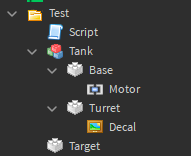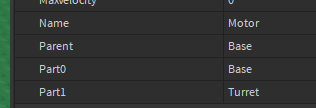# How do I make Motor6D joint look at Vector3 position?

Hello, I am trying to make `Motor6D` joint look at `Part`'s position which is `Vector3`.

Simple rotation such as the one below works like a charm.

``````turretMotor.C0 *=  CFrame.Angles(0, math.rad(90), 0)
``````

However, I need to make it look at a `Vector3` position. So I tried the following:

``````local target = script.Parent.Target
local motor = script.Parent.Tank.Base.Motor
local turretCF = motor.C0

while true do
-- Dont crash Roblox

-- C0 is in a object space so we should convert target position to object space as well?
local targetCF = target.CFrame:ToObjectSpace(turretCF)
motor.C0 = CFrame.lookAt(turretCF.Position, targetCF.Position) -- Update the C0
end
``````

StructureVideo

As you can see it doesn’t work. I would like to know why it doesn’t work and how to fix the issue. Thanks!

if the turret will stay there and not be moved then you can just anchor it and remove the motor6d. then you can do this:

``````motor = CFrame.new(turretCF.Position, targetCF.Position)
``````
1 Like

I understand, I know that, however, I am using Motor6D since I want it to work with moving assembly.

do you mean that their going to move around?

what is part1 and part0 of the motor6dthis should work

``````local target = script.Parent.Target
local motor = script.Parent.Tank.Base.Motor
local turretCF = motor.C0
local turret = script.Parent.Tank.Turret

while true do
-- Dont crash Roblox

-- C0 is in a object space so we should convert target position to object space as well?
motor.Part1 = nil
turret.CFrame = CFrame.new(turret.Position, target.Position)
motor.Part1 = turret
end
``````
1 Like

Now it does nothing, regardless of where I move the “target” part.

are there any errors? is the game even running?

There are no errors, and the game is indeed running.

try chinging this:

``````	motor.Part1 = nil
turret.CFrame = CFrame.new(turret.Position, target.Position)
motor.Part1 = turret
``````

to this:

``````	motor.Part0 = nil
turret.CFrame = CFrame.new(turret.Position, target.Position)
motor.Part0 = motor.Parent
``````

Now the turret is detached from the base. I don’t think that messing with Part0 and Part1 properties you will achieve anything not “hacky”.

yeah i guess your right lemme try finding soemthing else

Here my other attempts:

``````local function lookAt(base: CFrame, target: CFrame)
return (base - base.Position):Inverse() * CFrame.new(Vector3.zero) * CFrame.Angles(math.pi / 2, 0, 0)
end
``````
``````local function lookAt2(root: Part, origin: CFrame, target: CFrame)
local direction = (Vector3.new(target.Position.X, origin.Position.Y, target.Position.Z) - origin.Position).Unit
return CFrame.Angles(3 * math.pi/2, 0, math.pi) * CFrame.Angles(0, 0, -math.asin(direction.X)) * CFrame.Angles(0, 0, math.rad(-root.Orientation.Y)) * CFrame.Angles(-math.asin(direction.Y), 0, 0)
end
``````

Both didn’t work.

I got them from here:

i think i found something that works

1 Like